International Journal of Business and Economics Research
Volume 4, Issue 6, December 2015, Pages: 315-322

Vertical Competition and Outsourcing in a Supply Chain

Hans W. Gottinger

Strategy, Technology, Economics, STRATEC Consulting, Munich, Germany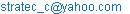Hans W. Gottinger. Vertical Competition and Outsourcing in a Supply Chain. International Journal of Business and Economics Research. Vol. 4, No. 6, 2015, pp. 315-322. doi: 10.11648/j.ijber.20150406.17

Abstract: This paper explores the importance of supply chain asymmetries by investigating the difference between firms in terms of key parameters. For the examination of inter-firm competition through product development, the context of simultaneous entry and sequential entry are treated separately. This allows a deeper understanding of the implications of information asymmetry and commitment which have been regarded as important determinants in the context of game-theoretic studies. The study of innovation based competition has often considered aspects related to patent races and incremental product-process innovation to achieve distinctive advantage. However, recently innovation-based competition has become an aspect of buyer-supplier relationships. There are many instances in manufacturing where one finds situations of lock-ins created by innovative suppliers.

Keywords: Supply Chain, Outsourcing, Competition, Investment Strategy, Stochastic Games

Contents

1. Introduction

The study of innovation based competition has often considered aspects related to patent racing and incremental product-process innovation to achieve distinctive advantage.

Lately innovation-based competition has also become an aspect of buyer-supplier.

Relationships, this appears very natural in view of the intrinsic relationship of networks, competition, innovation and industrial growth (Gottinger, 2016). There are many instances in manufacturing where one finds situations of lock-ins created by innovative suppliers. For example, in the computer industry Intel and Microsoft as suppliers of microprocessor and operating systems, respectively, to desktop manufacturers like IBM and Dell illustrate such innovation-based lock-ins. Indeed, there exists an evolving power structure (dubbed ‘channel power’) in a supply chain driven by innovation competence of its members. This paper focuses on innovation-based buyer-supplier competition as addressed strategically by Porter (1979). A business context is envisaged in which, at any given point in time of the relationship, both the buyer and the supplier could be pursuing innovation simultaneously. We recognize that the primary motivation for such investments in innovation by members of the supply chain is to increase their differential or relative channel power in the supply chain. Also in a situation where the buyer is locked in by a supplier, the buyer may actively pursue the creation of a substitute technology by investing in innovation. The primary motivation for the buyer in this case would be to eliminate the technology lock-in and become independent.

We can look at how three diverse companies view their value chain in their degree of vertical integration:

1)  Staples in the office products industry saw a multistep, high-cost value chain. They envisioned a future in which immense customer and shareholder value could be created by shortening the chain to capture margin while offering small businesses lower prices, wider product selection and more convenience. Staples enabled this value proposition with a model that supported its retail stores with its own central distribution centers. The centers required greater operational scale but allowed Staples to eliminate middlemen and increase margin.

2)  Consider how Intel developed its capabilities to sustain its growth path of innovation. Forced to exit the dynamic random access memory. (DRAM) chip market in the late 1980s by Japanese competitors, Intel knew it had industry-leading logic circuit design skills but had been outclassed on its manufacturing platform. Each of its chip fabrication plants, or ‘fabs’, was different, requiring different processes and a new learning curve at each fab when new products were rolled out. In response, the company launched a ‘copy exactly’ initiative to enable the transfer of new products and process flows into mass production with minimal variation.

3)  Another example is Starbucks’ actions to develop its capabilities in support of its path of channel innovation. The company has launched two initiatives: the first is to expand its food services account and the second to build its presence in grocery stores. To support the first initiative, the company has transitioned the majority of its food service accounts to broadline distribution networks and aligned its current food service sales, service and support resources with SYSCO Corporation, an established player in food service. To enable the second initiative, Starbucks is developing an alliance with Kraft Foods to market and distribute its whole bean and ground coffees to grocery stores.

In this context it boils down to how much integrated or disintegrated a company should become to maximize accumulated value in a value net. The Economist (2007) reports that the car industry is a case in point as an example of "the decomposition of the vertically integrated business model" from the one that used to be very integrated as part of a shift in a value net. In such circumstances, firms enter the crossroads of a very delicate strategic supply chain relationship. Specifically, a strategy ought to be in place to defend the ability to appropriate and accumulate value by ensuring that the suppliers of the resources that the firm chooses not to own are not able to put themselves in a position to leverage value to the firm. The PC industry provides an excellent example of power diffusion up the supply chain. In 1981, IBM designed product, process and supply chain such that it sourced the microprocessors from Intel and the operating system from Microsoft. The outcome was an outstanding successful product design but a disastrous supply chain design for IBM. Today, the power of Intel in the supply chain for PCs is undisputed but challenged by Advanced Micro Devices (AMD). The new innovations that occur in this industry are to a great extent defined by this upstream supplier of microprocessors. The lesson learnt is to beware of the ‘Intel Inside’ syndrome (Fine, 1999).

Extending this argument to the upstream microprocessor industry also provides some interesting observations. During the 1960s, the practice of second sourcing whereby innovative firms license production of one or more manufacturers that can act as a second source of any new product had already developed. It was alleged that some sole suppliers of semiconductors ‘exploited’ their customer firms once they had locked in their product designs to that of the supplier’s product. This feature of the industry profoundly affected the evolution of market structure, for it opened up a new and attractive strategy for second sourced suppliers. A firm would enter as a second sourcer and learn to produce high volumes efficiently while offering a leading edge product identical to that of the innovating firm. Once this hurdle was surmounted, it could use its growing cash flow to support a larger R&D effort with a view to developing its own next generation products. For example, AMD operated as a second sourcer in its early years, achieved considerable success, and later on more than half a dozen companies were second sourcing AMD’s product (Sutton, 2001).

An example can be found in the supply chain of automobiles (Clark and Fujimoto, 1991, Chaps.5, 6). Au­tomobile production begins with design, which consists of three main elements. The first element is the concept itself, thereafter, the design of the vehicle can be usefully divided into the macro-design (the development of the basic chassis, sub-assembly and component specification) and the micro-design (the development, to agreed specifications, of the vehicle's constituent components). The first two elements of design tend to be undertaken by the car assembler. In particular, the assembler takes charge of concept origination. In the face of intense competition, however, the costs associated with developing new vehicle prototypes have increasingly forced car assemblers to source the design of sub-assemblies and components (the micro-design) from external suppliers. The degree to which such outsourcing is undertaken by assemblers varies between different firms.

The assemblers who outsource the micro-design to external suppliers have a motivation to let these suppliers grow larger so that the supply bases can be brought up to global standards. These larger suppliers would then be required to take full responsibility for the design of sub-assemblies and for the coordination of the second and third-tier component manufacturers that contribute to the product.

The strong market position of these sub-assemblers is further enhanced by product specialization. No single supplier produces all types of sub-assembly. For example, Bosch, which is the world's largest automotive equipment manufacturer, targets its efforts on starter systems, spark plugs, braking systems, lighting and windscreen wipers.

The net result of market consolidation is that the supply of particular sub-assembly systems has become concentrated amongst just a handful of manufacturers.

Other examples in different industries abound in operations research studies on supply chains (Tang, C. S., Teo, C.-P. and K.K. Wei, 2008).

A differential game formulation, as applied to an innovation race (Gottinger, 2006) and high speed technological competition is used to examine the competition between the buyer and the supplier. Investigation of this type of buyer-supplier competition would lead to a better understanding of the dynamics of collaboration among supply chain partners.

An interesting aspect of the problem is the fact that the supplier must take into account the inherent incentives for the buyer to develop a ‘backstop’ technology which can be substituted for the supplied component. The supplier with the knowledge of this intent of the buyer acts such that the profits are maximized before the invention of substitute technology by the buyer. The time when such innovation materializes is uncertain, but can be affected by R&D efforts.

The model formulation is related to a case in the economics of exhaustible resources. Harris and Vickers (1995) analyze a dynamic game between a resource-exporting country and an importing country that is seeking to invent a substitute technology. They address a central question in the economics of exhaustible resources concerning incentives for the discovery of reproducible ‘backstop’ technologies to substitute for finite natural resources that are being depleted. Importing countries are motivated to discover backstop technologies not only to overcome the problem that resource stocks are finite, but also to reduce dependence upon resource producers, who often enjoy a considerable degree of monopoly power over them.

The organization of this paper is as follows. A stochastic differential game model for supply chain technological competition is presented in Section 2. In Section 3 the differential game is analyzed and the Markov-perfect Nash equilibrium results are given in Section 4. Section 5 provides a discussion of results. The proofs of theorems are provided in the Appendix.

2. Differential Game Formulation

The notations and symbols used in the model are as follows:

S: subscript for the supplier firm.

B: subscript for the buyer firm.

T: Finite time horizon for the strategies.

t: An instant of time in the dynamic game setup.

uB(t): Buyer's innovation effort (R&D expenditure per unit time).

uS(t): Supplier's production effort (Expenditure in production processes and resources per unit time).

p(uS): Price/ Inverse demand curve.

cS(uS): unit production cost to be incurred by the supplying firm.

cB: unit production cost to be incurred by the buying firm (Assumption cS >cB).

cU(uB): Effort cost incurred by the buying firm for achieving the level of inno­vation that would be a perfect substitute for the supplier S's innovation. τ

τ: random instant of time at which the level of innovation (breakthrough inno­vation) is reached.

F(t): the probability that the buying firm B is successful in the innovation project by time t, that is Pr(τ < t) = F(t).

k01: function representing the switching rate.

x(t): state dynamics represented by remaining demand for the product.

The context of buyer-supplier relationship that is based on innovation and quality parameters can be considered in the broad rubric of a resource-based view of a firm. Specifically, the vendor S is providing a resource in the form of innovation capabilities to the buyer firm B. There is a fixed known demand for the product. A one- to- one correspondence is considered such that for every product sold by the buyer to the final consumer, at least one component is required from the supplier. The supplier is assumed to be a monopolist and the buyer faces perfect competition in the final consumer market.

It is asserted that a one – to - one correspondence assumption helps in the analysis in two distinct ways. First, this allows explicit examination of dependence between the two collaborating partners and the evolution of power structure based on innovation. Second, this lends analytical simplification for solving the problem. The supplier S is a monopoly producer of a product component for which the cost of production is cS(uS) > 0 per unit. Supplier S's rate of production is a function of production effort uS and is assumed to be γuS. For analytical simplicity γ is assumed to be equal to 1. The rate of production is defined as, uS: (0,) [0, uS] The remaining market demand (measured as a percent of initial demand xo) is the state variable for the problem. In the context of the model specification and the underlying assumptions, the remaining demand x is identical for the buyer and the supplier. This remaining demand, x, is assumed to be a function of the supplier's production rate and the rate of change of remaining demand is,

x(t) = - γ uS (x(t)) = -uS (x(t)); x = xo.

As the production rate increases, the remaining market demand falls more rapidly. The price function of the buyer firm B, p(uS) is a function of the production rate of the supplier. The buyer invests to create a substitute technology which is assumed to enable production of the product component at a unit cost cB ≤ cS. In the event of a successful creation of such a technology there will be no demand for the supplier's component. The investment policy in innovation chosen by B is denoted by uB: (0, ) [0, ). This investment represents the buyer's efforts in R&D and is a function of the remaining demand x(t). By investing in innovation, the buyer gains knowledge and the time path for the buyer's level of knowledge k(t) is given by,

k(t) =uB (x(t)), k(0) = 0.

The outcome of innovation investments is considered to be uncertain. Therefore, we assume that the R&D investments don't precisely determine the date of success of innovation. A natural and convenient way to represent uncertain innovation is to suppose that the instantaneous probability of the buyer B innovating, conditional on not having so far done so, is a function of its current R&D effort rate. This ‘hazard rate’ approach, which derives from an exponential probability distribution, has been used by numerous authors, e.g. Dasgupta and Stiglitz (1981), Harris and Vickers (1995). The probability that the buyer B has innovated by t is assumed to take an exponential form, which is independent of the accumulated knowledge level, k(t).

F(t) / [1 – F(t)] = uB (x(t))    (1)

where F(t) is the probability that the buyer B is successful in the innovation project by time t; that is Pr(τ < t) = F(t).

Let π(uS) = [p(uS) cS(uS)] γuS be the supplier's instantaneous profit function. This instantaneous profit function acts as the objective functional for the supplier. In the problem context, the buyer faces perfect competition. The buyer invests in innova­tion to become independent of the lock-in created by the supplier. Since the buyer is facing perfect competition, it maximizes consumer surplus. A figure representing the consumer surplus is provided in figure 1. Let the consumer surplus be denoted by σ(uS) = 0uS p(y))dy – uSp(uS). After the successful invention of substitute tech­nology, a new market is created for the buyer's product. Now, the buyer can gain discounted profits depending upon the price pB(uB) and the variable cost of produc­tion cB(uB) The payoff of the buyer firm B is 0τ e-rt [s(uS(x(t))) - cU(uB(x(t)))]dt+ τ e-rt πB (uB)dt, where cU is the buyer's cost of innovation effort, τ is the random time at which innovation occurs, r > 0 is the discount rate, πB is the price that the buyer can charge for the product once the innovation materializes and πB (uB) is the profit earned by the buyer after time τ. It is a function of the price charged and the variable cost of production. The payoff of the supplier firm S is 0τ e-rt π(uS(x(t)))dt.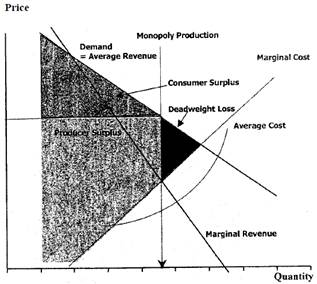Figure 1. Monopoly Production and Price.

The strategies uS(.) and uB(.) constitute an equilibrium if and only if, for all x0 > 0, taking uB(.) as given, the production effort uS(.) maximizes the supplier's payoff among all remaining strategies; and similarly, taking uS(.) as given, the innovation effort uB(.) maximizes the buyer’ s payoff among all possible strategies. The following assumptions are considered for the inverse demand function, the profit function and the cost of effort function.

(i) The inverse demand function p(uS) is twice continuously differentiable on [0,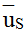]:

p´< 0 on this interval; p(0) > cS 0; and p () = 0. This assumption signifies that there is a finite price p(0) to sustain a profitable production, the inverse demand function is downward sloping, and that the total demand for the supplier's output at any given time is bounded by the maximum possible production rate(ii) The profit function π (uS) is strictly concave on [0,]: π" < 0, which is equiv­alent to

2p' + p"uS < 0.

(iii) The cost of production effort cS(uS) is continuously differentiable on [0,): cS(0) = 0 on [0,) and c'S(y) is a constant. The cost of innovation effort cU(uB) is twice continuously differentiable on [0, ); cU (0) = c´U (0)  = 0; c²U > 0 on [0, ); and c´U (y) as y . This assumption suggests that costs increase and are strictly convex in effort. It also suggests that zero R&D effort is suboptimal and for the strategy uB we can confine our interest to interior solutions.

In this market setting, it is assumed that the product enjoys a very large fixed demand. Furthermore, whatever is produced by the supplier gets sold and hence the production rate of the supplier equals the quantity demanded per unit time in the market. The inverse demand function or in other words the price charged by the supplier is conceptualized as a linear decreasing function of units produced per unit time.

p(uS(x(t)))=a-bγuS(t)=a–buS(t); a>cS>0, b>0 are constants (2)

The variable cost of production is assumed to be linear and an increasing function of production effort uS.

cS = c1 [uS], c1 > 0 is a proportionality constant   (3)

The cost of effort function for the buyer is assumed to be strictly convex and increasing in innovation effort uB.

cU = c2 [uB]2, c2 > 0 is a proportionality constant (4)

Note that this problem has the same state dynamics for the buyer and the sup­plier. The objective functional of supplier S is the expected profit until the random instant of time τ,

JS(x) = EuB(.)0τ e-rt [p(uS(x(t))) cS(uS)]γuS x(t))]dt= EuB(.)0τ e-rt [a - buS(x(t)) - c1(uS)(x(t))uS( x(t))] dt (5)

Note that the supplier S receives zero profit for t > τ (since demand vanishes). The objective functional of firm B is the expected gain from innovation efforts, given by

JB(x, uB) = EuB(.) 0τ e-rt[s(uS(x(t))) cU(uB)(x(t)))]dt + τ e-rt πB (uB)dt= EuB(.)0τ e-rt [s(uS(x(t))) c2(uB)(x(t)))]2dt + τ e-rt πB (uB)dt    (6)

where

s(uS(x(t))) = 0uS [p(y)dyuS (x(t))p(uS ) = 0uS [a - by]dy -uS(x(t)) [a - buS(x(t))]   (7)

is the surplus gained by the customers if the buyer firm B is able to accomplish the required innovation level.

The problem formulation for the supplier is:

JS(x) = EuB(.)0t e-rt [p(uS(x(t))) cS(uS)]uSx(t))]dt= EuB(.)0t e-rt [a - buS(x(t)) - c1(uS)(x(t))]uS( x(t))dtsubject to x(t) = -uS (x(t)); x(0) = xo(8)

The problem formulation for the buyer is,

JB(x,uB) = EuB(.)0τ e-rt [s(uS(x(t)))cU(uB)(x(t)))]dt + τ e-rt πB (uB)dt= EuB(.)0τ e-rt [s(uS(x(t))) c2(uB)(x(t)))]2dt + τ e-rt πB (uB)dt

subject to x(t) = - uS (x(t)); x(0) = xo (9)

The differential game defined by (8) and (9) belongs to the class of piecewise deterministic games (Dockner et al., 2000): The game has two modes only: mode 0 is active before firm B has made the innovation and mode 1 becomes active if firm B succeeds in making the substitute technology. Thus, there can be at most one switch of mode. The switching time is the random variable τ, and the probability distribution of τ is F, as given in equation (1).

3. Analysis of the Model

The model is an extension of a previous model and results as discussed in Gottinger (2015).

The variables are written without the arguments for notational convenience. For example, uS (x (t)) = uS. In the game specified in equation (8) and equation (9), the nature of stationary Markov perfect Nash equilibrium conditions for the supplier and buyer are analyzed. Supplier S's stationary Markovian strategy for production effort US (h, x) is a mapping US: M ´(0, ) [0,], where M= {0, 1} is the set of modes. Buyer B's stationary Markovian R&D effort strategy UB ( h, x) is a mapping UB: M ´(0, ) [0, ).

The solution approach in Harris and Vickers (1995) is adopted and is presented below.

Let the value functions of the supplier and buyer be defined as VS(h,s) and VB(h,s) respectively for h M = {0, 1} and x (0, 1). As required from the def­inition and theorem pertaining to piecewise deterministic differential games provided in the Appendix, these value functions are assumed to be bounded and continuously differentiable and for all x [0, l] that satisfy the Hamilton-Jacobi-Bellman (HJB) equations.

rVS(0,x) = max{π(uS) - uSVxS(0,x) + UB(0,x)[VS(1,x) - VS(0,x)]½ uS [0,]}  (10)

rVS(1,x) = 0    (11)

rVB(0,x) = max{s(US(0,x) - cu(uB) - US(0,x)VxB(0,x) + uB [VxB(1,x) - VxB(0,x)]½uB [0,]}    (12)

rVB(1,x) = π(uB)    (13)

Equations (11) and (13) present the value functions for the supplier and the buyer once the system switches to mode 1. As reasoned earlier, the supplier's profit stream becomes zero after the switch as there will be no demand for supplier's product component. On the other hand, in the event of being successful in making the innovation; the buyer earns the present value of the constant stream of consumer surplus over an infinite interval of time. Additionally, irrespective of the system mode, it can be observed that the supplier's profit will become zero if there is no remaining demand. In the absence of any production by the supplier, it is assumed that the buyer gets a constant expected payoff VB which is the optimal value of a one player stochastic control problem with buyer B as the decision maker. Hence, the value functions are subjected to two constraints,

VS(h,0) = 0, " h   (14)

VB(0.0) =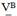The maximizing sets of uS [0,] and uB [0,) that maximize the respective value functions of the supplier and the buyer in equation (10) and (12) are denoted as FS(x) and FB(x), respectively. If US(0,x) FS(x) and UB(0,x) FB(x) for all x [0,1],and provided that the value functions can be found that satisfy HJB equations; then from the theorems on Markov games (Dockner et al., 2000), the strategy pair (US( h, x), UB (h, x))is a stationary Markov perfect Nash equilibrium. To determine the value functions, the equations (10) and (12) can be rewritten as,

rVS(0,x) = GS (VxS(0,x))-UB(0,x) VS(0,x)    (15)

rVB(0,x)=s(US(0,x))-US(0,x)VxB(0,x)+GB[`VB-VB(0,x)] (16)

where,

GS(z) = max {π(uS) - uSz½0 £ uS £};

GB(z) = max {zuB - cU(uB)½0 £ uB}

US(0,x)) = argmax {π(uS) - uS VxS(0,x)½ 0 £ uS £}  (17)

UB(0,x) = argmax {[VB - VB(0,x)] uB - cU(uB)½ 0 £ uB} (18)

Solution of the system of autonomous nonlinear differential equations (15) - (18) leads to the determination of the two value functions. Substituting the expressions for the demand function and the cost of effort function from equations (2) and equation (4), respectively.

GS(z) = max {a - buS - c1 uS ] uS - uS z½0 £ uS £}  (19)

GB(z) = max {zuB - c2(uB)2½0 £ uB }    (20)

US(0,x)) = argmax{a-buS-c1 uS]uS-uS VxS(0,x)½0£uS £} (21)

UB(0,x) = argmax {[`VB - VB(0,x)] uB - c2(uB)2½ 0 £ uB}(22)

where,

VB = π(uB) /r    (23)

4. Equilibrium Results

Theorem 1. If VS* and VB* denote the positive equilibrium value of the supplier and buyer firm, then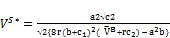(24)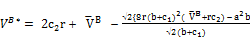(25)

constitute the equilibrium pair.

Theorem 2. If US* and UB* denote the positive equilibrium control functions of the supplier and buyer firm, then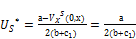(26)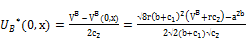(27)

Theorem 3. At equilibrium the remaining demand at time t can be expressed as: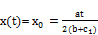(28)

where x(0) = xo is the initial remaining market demand.

5. Observations and Discussion

Observation 1 At equilibrium the supplier chooses a monopoly production rate.

The observation can be explained by exploring the monopoly production rate of the supplier in the given setting. The monopoly profit of the supplier πm is

πm = max {π(uS)½0 £ uS £} = max {[a - buS - c1 (uS)] uS ½ uS [0,]}

The corresponding production effort required to attain a monopoly profit is therefore,

uSm = argmax { π(uS)½0 £ uS £} = argmax {a - buS - c1( uS) ] uS ½ uS [0,]}

Taking the first order condition leads to the monopoly production rate given by a uSm = a/ 2(b+c1).

The supplier is assumed to be a monopoly. In the given setup the supplier employs monopoly production rate to gain as much of monopoly profits as possible before being locked out of the market. An illustration of such a monopoly production is provided in figure 2.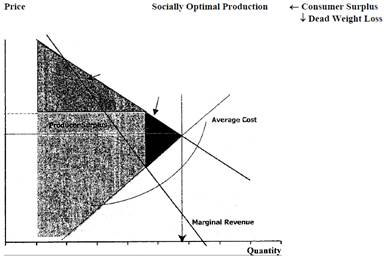Figure. 2. Price Range.

At equilibrium the supplier exercises monopoly power and allows the buyer to pursue creation of substitute technology. The supplier sets the monopoly production rate at the start of the game and it remains time invariant for the entire planning horizon. Next, the observation regarding innovation effort of the buyer is presented.

Observation 2 (i) The innovation effort of the buyer increases with the potential value of time discounted profit `VB that the buyer can receive in the event of successful creation of substitute technology. (ii) The monopoly production rate of the supplier exerts a negative effect on the buyer's innovation rate.

The observation stated in 2(i) is intuitive and can be observed from the expression for buyer's equilibrium control given in equation (27). The motivation for the supplier to invest in substitute technology would indeed be dependent on the discounted profits that the buyer can obtain in case the innovation is successful. The time of successful `invention' is uncertain but it can be influenced by the buyer's innovation efforts. This is expressed in equation (1). For observation 2(ii) the expression for equilibrium innovation rate by the buyer UB* is revisited. With some algebraic manipulations the equilibrium innovation rate of the buyer can be expressed as:

UB* = `[(VB + rc2)/ c2) - (US*)2/2 c2] -  (29)

From the above expression it can be noted that the supplier's monopoly production rate exerts a negative effect on the buyer's innovation efforts. Observation 1 and 2 characterize the nature of equilibrium control of the buyer and the supplier. Indeed, in the given setting there exists no motivation for the two players to collaborate and each acts in its best interest. The supplier makes full use of the monopoly power and the buyer continues investments in innovation to create substitute technology.

However, as asserted by Schelling (1980, p.4), if we confine our study to the theory of strategy, we seriously restrict ourselves by the assumption of rational behavior. The result obtained doesn't necessarily suggest ‘intelligent’ behavior but the equilibrium results are suggestive of a behaviour motivated by a conscious calculation of advantages, a calculation that in turn is based on explicit and internally consistent value systems. Still, the assumption of rational behavior is a productive one as it gives us a grip on the subject. In the problem context the availability of equilibrium solution based on the assumption of rationality permits us to identify our own analytical processes with those of the hypothetical participants in a conflict. By further investigation of a certain kind of consisterrcy in the behavior of our hypothetical participants, we can examine alternate courses of behavior according to whether or not they meet those standards of consistency.

The equilibrium control of the buyer and the supplier presents a dilemma in achieving a long-term collaborative supply chain partnership. The solution for this dilemma for both firms is to create close ties with one another so as to minimize the risk of opportunism. These ties should deter the supplier from using monopoly power aud the buyer from investing in substitute technology. The solution is to build credible commitments into the relationship. The next observation presents insights for building such credible commitments.

Observation 3 At equilibrium if the supplier's production rate is such hat US* = 2/b[(r`VB + c2)/ c2) - r2)] then the buyer sets its innovation effort to zero.

Analytically, the above expression can be obtained by setting UB* = 0 in equation (29) and some algebraic manipulations. Note, that in equation (29) we require (VB + rc2)/ c2 > (US*)2/2 c2 to ensure a real value of the expression. Moreover, it is also intuitive to note that the only way the supplier can make the buyer set its innovation efforts to zero is by increasing the production rate It can be observed from figure 2 that with a downward sloping demand curve, the price charged by the supplier decreases with an increase in its production. The inverse demand curve (price) of the supplier's product component is (a – buS). The monopoly price charged by the supplier is therefore p* = a -b[a/ 2(b+c1)].

With the changed production rate, the price becomes,

`p = a -b[ 2/b[(r`VB + c2)/ c2) - r2)]]  (30)

The observation suggests that there is a price bandwidth that the supplier can credibly commit to the buyer and achieve a long-term partnership. It can be reasonably asserted that based on the cost structures there is a p below which the supplier makes losses. Theoretically, at this price marginal cost equals average revenue. Therefore the price range that a supplier can charge and achieve a long-term relationship with the buyer can be given as p < p< `p. With this price range the supplier can create a disincentive for the buyer to invest in substitute technology. In the problem context considered in this paper, the motivation for the supplier to adopt such a policy depends on two considerations. First, the price should be at least high enough to cover marginal costs. Second, if the supplier wishes to remove uncertainty in the relationship and intends to be the preferred partner for the entire planning horizon, the supplier will adopt this policy. Particularly the second consideration depends on the profits that can be earned for the time period [0, τ] using a monopoly pricing with the profits that can be earned for the entire planning horizon [0, ) by judiciously choosing a price from the given range. The choice of price from the given range would be such that the supplier starts with a price p= `p and gradually reduces it over time till it reaches p.

It is important to note that in the problem context, time τ is not deterministic and is characterized by the hazard rate. The time instant τ could approach or alternately it could be very close to 0, depending on the outcome of innovation investments by the buyer. The pricing scheme obtained from this analysis provides implications for the supplier to create conditions for trust-based governance. The formation of buyer-supplier trust emanates from certain proactive measures taken by the collaborating partners as a part of their contract. Cox et al. (2002, p. 14) suggest some other aspects for forming long-term relationships. To avoid potential conflict between a, buyer and a supplier due to relative difference in innovation competence, one approach is to have either the buyer or the supplier make dedicated investments. The other partner could protect its interest by posting a bond that it would forfeit if the partner investing in innovation acts opportunistically. The authors assert. "This bond, which is known in transaction cost parlance as a hostage, would be used to create a condition of bilateral dependency (more commonly referred to as "interdependency"). Yet another approach to create conditions for interdependency is that both the collaborating partners invest in innovation. Such joint innovation activity strengthens the bond between collaborating firms and enables long-term relationship formation.

Appendix

Proof of Theorem 1.

From equations (19), (20), (21) and (22), the maximization of the expression in the right hand side leads to the expressions,

GS(z) = (a - z)2 / 4(b + c1  (A.1)

GB(z) = z2 / 4c2    (A.2)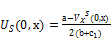(A.3)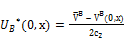(A.4)

with `VB as a given pre-equilibrium level.

The system of autonomous nonlinear differential equations as specified in equations (15) and (16) can be analyzed by exploring the corresponding equilibrium points or critical points of the equations. These points are obtained by setting VxS = 0 and VxB = 0, respectively.

Equations (15) and (16) thus reduces to,

rVS(0,x) = GS (0) -UB(0,x) VS(0,x)    (A.5)

And

rVB(0,x) = s(US(0,x)) + GB [`VB - VB(0,x)]    (A.6)

Substituting for GS, GB, US(0,x), UB(0,x) from equations (A.1) – (A.4), and solving for S(0,x) and VB(0,x) leads to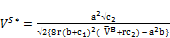(A.7)

And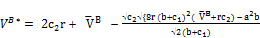(A.8)

Proof of Theorem 2.

The equilibrium value of US*(0,x) is obtained by substituting VxS(0,x) = 0 and finding the argument uS that maximizes the right hand side of equation (21). Similarly, the equilibrium value UB*(0,x) is obtained by finding the argument uB that maximizes the right hand side of equation (22) and substituting the equilibrium point VB*(0,x) from equation (25). Therefore, (26) and (27) follow.

Proof of Theorem 3.

The theorem follows by substitution of uS = US* in the equation for state dynamics and solving the resulting first order differential equation

x(t) = - US* = - a/2(b+c1) which leads to x(t)=xo-at/2(b+c1)

where x(0) = xo is the initial remaining market demand.

References

1. Clark,K.B. and Fujimoto,T. (1991), Product Development Performance, Boston, Ma.: Harvard Business School Press.
2. Cox, Andrew; Ireland, P. Lonsdale,C.Sanderson,J.;Watson,G.(2002), Supply Chains, Markets and Power: Mapping Buyer and Supplier Power Regimes,London: Routledge.
3. Dasgupta,P., Stiglitz,J.(1981),"Resource Depletion under Technological Uncer­taintv",Econometrica49, 85-104.
4. Dockner,E., Jorgensen,S., Van Long, N., Sorger, G (2000), Differential Games in Economics and Management Science, Cambridge: Cambridge Univ. Press.
5. The Economist (2007), "Revving Up", A Special Report on Innovation, Oct.27, 6-10.
6. Fine,C,H, (1999), Clockspeed: Winning Industry Control in the Age of Temporary Advantage, New York: Perseus.
7. Gottinger,H.W. (2006), Innovation, Technology and Hypercompetition, London: Routledge.
8. Gottinger,H.W. (2015), "Supply Chain Coopetition", International Journal of Business and Economics Research 4(2),67-71.
9. Gottinger,H.W.(2016), Networks,Competition, Innovation and Industrial Growth, NovaScience: New York.
10. Harris, C., and Vickers,J.(1995),"Innovation and Natural Resources: A Dynamic Game with Uncertainty",The Rand Journal of Economics26(3), 418-430.
11. Porter,Michael E.(1979), "How Competitive Forces shape Strategy", Chapter 1 inOn Competition, Boston: Harvard Business School Press, 21-38.
12. Schelling, T. (1980), Strategy and Conflict, Cambridge,Ma.: Harvard Univ. Press.
13. Sutton, J.(2001), Technology and Market Structure, MIT Press: Cambridge,Ma.
14. Tang,C.S., Teo,C.-P. and K.K. Wei, eds. (2008), Supply Chain Analysis, Springer: New York.

 Contents 1. 2. 3. 4. 5.
Article ToolsAbstractPDF(1857K)